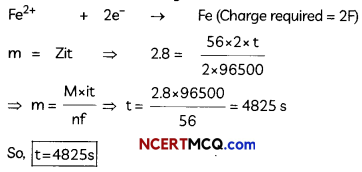Students can access the CBSE Sample Papers for Class 12 Chemistry with Solutions and marking scheme Term 2 Set 2 will help students in understanding the difficulty level of the exam.

## CBSE Sample Papers for Class 12 Chemistry Term 2 Set 2 with Solutions

Time Allowed: 2 Hours
Maximum Marks: 35

General Instructions:

• There are 12 questions in this question paper with internal choice.
• SECTION A – Q. No. 1 to 3 are very short answer questions carrying 2 marks each.
• SECTION B – Q. No. 4 to 11 are short answer questions carrying 3 marks each.
• SECTION C- Q. No. 12 is case based question carrying 5 marks.
• All questions are compulsory.
• Use of log tables and calculators is not allowed.

Section – A
(Section A-Question No 1 to 3 are very short answer questions carrying 2 marks each.)

Question 1.
(A) Arrange the following amines in order of decreasing basic strength—Ethylamine, ammonia, tri ethylamine.
Triethyl amine (C2H5)3N> Ethyl amine C2H5NH2> Ammonia NH3
Explanation: More the number of alkyl groups, more the +1 effect so more the basic strength.

(B) Why aniline is acetylated first to prepare mono bromo derivative ? (2)
Due to the strong activating effect of amino group, aromatic amines readily undergo electrophilic substitution reactions and it is difficult to stop the reaction the monosubstitution stage. Therefore, the acetyl group attached with aniline deactivates the aniline due to its electron withdrawing effect. Thus acetylation of aniline reduces its activation effect.

Question 2.
If the half-life period of a first-order reaction in A is 2 minutes, how long will it take to reach 25% of its initial concentration? (2)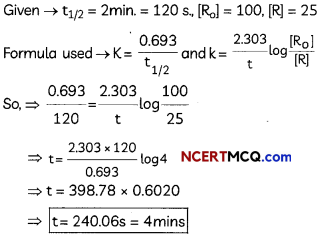Question 3.
Draw the structure of the alcohol that could be oxidized to each compound. (2)
(i) 2-propanone
(ii) Cyclohexanone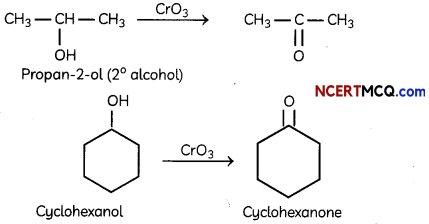Section – B
(Section B-Question No 4 to 11 are short answer questions carrying 3 marks each.)

Question 4.
Using thermodynamic principles, explain: (3)
(A) Entropy has negative value for adsorption.
The randomness of the overall process decreases due to the presence of a force of attraction between adsorbent and adsorbate due to which the gas molecules stick to the surface. So, Entropy has negative value for adsorption.

(B) Adsorption is a exothermic process?
Adsorption is an exothermic process since surface particles of the adsorbent are unstable and when the adsorbate is adsorbed on the surface, the energy of adsorbent decreases, and this results in the evolution of heat. Therefore, adsorption is always exothermic.

(C) Relation between enthalpy and entropy at equilibrium.
When ΔG becomes zero, adsorption equilibrium is established. Since it is an exothermic process, ΔH is negative. Since the adhering of gas molecules to the surface lowers the randomness, the ΔS is negative. So, ΔH and TΔS become equal at equilibrium to make ΔG zero. (ΔG = ΔH – TΔS)Question 5.
The following table summarizes the oxidation states of the 3d series elements.Account for the following questions related to the above table:
(A) Transition metals show variable oxidation state.
(B) At the beginning of the series, +3 oxidation state is stable but towards the end +2 oxidation state is stable.
(C) Mn has six different oxidation states from +2 to +7.
OR
Explain the trends in the properties of the members of the First series of transition elements:
(A) E0 (M2+/M) value for copper is positive (+0.34V)in contrast to the other members of the series.
(B) Cr2+ is reducing while Mn2+ is oxidising though both have d4 configuration.
(C) The oxidising power in the series increases in the order VO2+ < Cr2 O72- < MnO4 (3)
(A) Transition elements show variable oxidation states because their valence electrons are in two different sets of orbitals, that is (n-l)d and ns. The energy difference between these orbitals is very less, so both the energy levels can be used for bond formation. Thus, transition elements have variable oxidation states.

(B) The number of oxidation states increases with the number of electrons available, and it decreases as the number of paired electrons increases.
Explanation: In 3d series, first element Sc has only one oxidation state +3; the middle element Mn has six different oxidation states from + 2 to +7 because number of unpaired electrons is maximum but the last element Cu shows +1 and +2 oxidation states only because it has maximum number of paired electrons.

(C) The different oxidation states of manganese (Mn) are +2, +3, +4, +5, +6 and +7. Manganese with 3d5, 4s2 as the outer electronic configuration, shows oxidation states ranging from +2(3d5) to + 7(3d0). The +2 oxidation state is very stable due to exactly half-filled 3d-orbital of higher stability.
OR
(A) Copper has high atomisation ΔaH° and low hydration energy ΔhydH°. Due to which the E° value is positive.
Explanation: The Eθ(M2+/M) value of a metal depends on the energy changes involved in the following:
1. Sublimation : The energy required for converting one mole of an atom from the solid state to the gaseous state.
M(x) → M(g) Δs H(Sublimation energy)

2. Ionization : The energy required to take out electrons from one mole of atoms in the gaseous state.
M(x) → M(g) Δi H(lonization energy)

3. Hydration: The energy released when one mole of ions hydrated.
M(g) → M2+(ag) Δhyd H(Hydration energy)
Now, copper has a high energy of atomization and low hydration energy. Hence, the EΦ (M2+/M) value for copper is positive.

(B) Cr2+ is reducing agent as its configuration changes from d4 to d3, when it is oxidized to Cr3+ . On the other hand, the reduction of Mn3+ to Mn2+ results in the half-filled (d5) configuration which has extra stability hence Mn3+ acts as oxidizing agent.

(C) Greater the oxidation state, higher is the oxidising power.
Explanation: Oxidation state of V in VO2++ is 5, Cr in Cr2O72- is 6 and Mn in MnO4 is 7.Question 6.
Based on valence bond theory, explain the bonding in the coordination entity [Co(NH3)6]3+. Also, comment on the geometry and spin of the given entity. (Atomic no. of Co = 27) (3)
[Co(NH3)63+
Co3+ = 3d6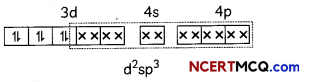In this complexion, the oxidation state of cobalt is +3.
This complex involves the d2sp3 hybridisation. The six pairs of electrons, one from each NH3 molecule, occupy the six hybrid orbitals. It proves that complex has octahedral geometry.

Question 7.
A metal complex having composition Cr(NH3)4Cl2Br has been isolated in two forms A and B. The form A reacts with AgN03 to give a white precipitate readily soluble in dilute aqueous ammonia, whereas B gives a pale-yellow precipitate soluble in concentrated ammonia
(i) Write the formulae of isomer A and B
(ii) State the hybridisation of Cr in each of them.
OR
Give reason for each of the following:
(A) Co2+ is easily oxidised to Co3+ in presence of a strong ligand.
(B) CO is a stronger complexing reagent than NH3.
(C) The molecular shape of Ni(CO)4 is not the same as that of [Ni(CN)4]2- (3)
(i) Formula of A → [Cr(NH3)4 Br Cl] Cl
Formula of B →[Cr(NH3)4 Cl2] Br
Explanation: ‘A’ and ‘B’ are Ionisation isomers. ‘A’ has Cl as onion so it gives white ppt. with AgNO3
Whereas ‘B’ has Br as onion so it gives pale yellow ppt. with AgNO3.
(ii) Hybridisation state of Cr in each of them is d2sp3.
OR
(A) Co2+ ions can be easily oxidised to Co3+ ions because the crystal field stabilisation energy of Co3+ ions with a d6 configuration is higher than d7 configuration.
In presence of strong ligand, the d- orbitals of metal gets splits up into two set of orbitals that is t2g and eg. The half filled or fully filled t2g is more stable than other configuration. This is because it gives maximum amount of low energy of t2g orbital with electrons. Lower the energy, more is the stabilisation.

(B) Since CO can form a as well as n bond, whereas NH3 has lone pair of electrons and can form a bond only. Therefore, CO is a better complexing reagent than NH3.
Explanation: CO has empty π orbitals which overlap with filled d-orbitals (t2g orbitals) of transition metals and form π bonds by back bonding. These π interaction increases the value of crystal field stabilisation energy (∆0).
As NH3 cannot from π bonds by back bonding, therefore, CO is a stronger ligand than NH3.

(C) In [Ni(CO)4], nickel is present in zero oxidation state {Ni = 3d8 4s2} but in [Ni(CN)4 ]2-, oxidation state of Nickel is +2. So, Ni2+: 3d8 4s0.
Explanation: Electronic configuration of:
Ni [Ar]4s2, 3d8
So Ni (0): [Ar] 4s0, 3d10
CO ligand causes pairing of electrons and shifting of electrons from 4s to 3d.
[Ni(CO)4]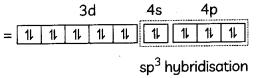[Ni(CO)4] has sp3 hybridisation and has tetrahedral shape.[Ni (CN4)]2- has dsp2 hybridisation and has square planar shape.Question 8.
The graphical representation of concentration of A Vs time is given for a general A → B,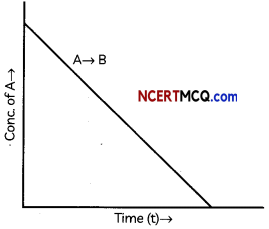The following questions:
(a) What is the order of the reaction?
(b) What is the slope of the curve?
(c) State the units for the rate constant. (3)
(A) Zero order reaction
(B) slope = – k
(C) Unit of k = mol L-1 s-1

Explanation: In case of a zero-order reaction, the rate of reaction is independent of the concentration of the reactant. The concentration [A]t of the reactant at a time t is given by [A]t = – kt + [A]0 (y = – mx +c) where [A]0 is the initial concentration of the reactant and k is a rate constant.
When the concentration of the reactant is plotted against time, a straight line with the slope equal to – k is obtained.

Question 9.
Complete the following reactions: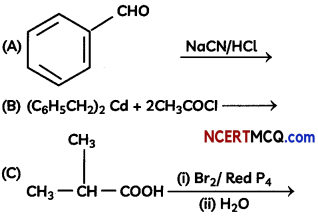OR
Write chemical equations for the following reactions:
(A) Propanone is treated with diLute Ba(OH)2.
(B) Acetophenone is treated with Zn(Hg)/ Conc. HCI
(C) Benzoyl chloride is hydrogenated in presence of Pd/BaSO4. (3)Explanation: Reaction is Hell-Vollard-Zelinsky reaction in which carboxylic acids react with chlorine or bromine in presence of small amount of red phosphorus to give a-halocarboxylic acids.
OR
(A)Explanation: Aldol condensation is an organic reaction in which an enolate ion reacts with carboxyl compound to form β-hydroxy aldehyde or β-hydroxy ketone. Hydroxide functions as a base and therefore moves the acidic a-hydrogen producing the reactive enolate ion.

(B)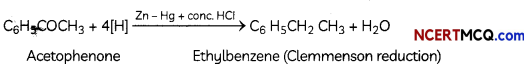Explanation: The Clemmensen reduction is a reaction that is used to reduce aldehydes or ketones to alkanes using hydrochloric acid and zinc amalgam.

(C)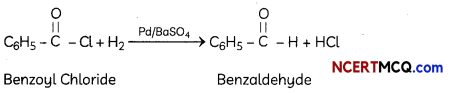Explanation: Rosenmund reduction is the hydrogenation of an acyl chloride to an aldehyde, in presence of catalyst (Pd supported on barium sulphate). Barium sulphate partially poisons Pd metal and prevents over-reduction. Untreated Pd catalyst is too reactive.

Question 10.
(A) Account for the following:
(i) Cl- CH2COOH is o stronger acid than CH3COOH.
(i) Cl group is an electron withdrawing creating less electron density on oxygen of carboxylic acid making the release of proton easier than acetate ion. Hence, Cl-CH2COOH is a stronger acid than CH3COOH.

Explanation: Chlorine due to its -1 effect increases the acidity of carboxylic acids by stabilising the conjugate base due to delocoalisation of the negative charge by resonance effect.

(ii) Carboxylic acids do not give reactions of the carbonyl group.
Carboxylic acids do not give characteristic reaction of carbonyl group. This is because the lone pairs on oxygen atom attached to hydrogen atom in the -COOH group are involved in resonance thereby making the carbon atom less electrophilic Hence, carboxylic acids do not give characteristic reaction of carbonyl group.

Explanation: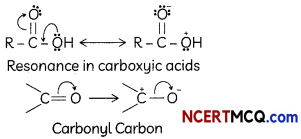(B) Give simple chemical tests to distinguish Benzoic acid and Phenol. (3)
Phenol and benzoic acid can be distinguished by ferric chloride test.
Ferric chloride test: Phenol reacts with neutral Fecl3 to form an iron-phenol complex giving violet colouration. But benzoic acid reacts with neutral FeCl3 to give a buff coloured ppt.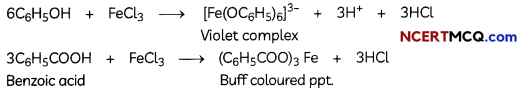Question 11.
(A) Aniline is a weaker base than cyclohexylamine. Give reason.
(B) Amino group in aniline is ortho and para directing, Then why does aniline on nitration give substantial amount of m-nitroaniline?
(C) There are two test tubes (abetted A and B. Give suitable test to identify which test tube contains aniline and which contains methylamine.
OR
(A) Arrange the following in increasing order of their pKa values:
(i) C6H5NH2, C2H5NH2, (C2H5) 2NH, NH3
(B) Identify the compounds A, B, C and D In the following set of reactions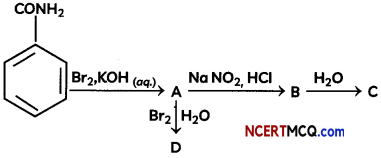(A) In aniline, benzene group is electron withdrawing in nature. Hence, it decreases the availability of electron pair on N-atom and is less basic. On the other hand, in cyclohexylamine, the cyclohexyl group is electron releasing in nature. Hence, this, increases the availability of electrons on N-atom and is more basic.

(B) Nitration is carried out in an acidic medium. In an acidic medium, aniline is protonated to give anilinium ion (which is meta-directing). Due to which aniline on nitration gives a substantial amount of m-nitroaniline.

(C) Nitrous acid Test – Treat aniline and methylamine with sodium nitrite with hydrochloric acid at zero degree Celsius.
In case of aniline: stable diazonium salt will be formed which will give phenol on hydrolysis.
In case of methylamine: unstable diazonium salt of aliphatic amine will be formed which will further get decompose into bubbles of nitrogen gas and methanol.
OR
(A) Increasing order of pKa value-
(C2H5)2NH < C2H5NH2 < NH3 < C6H5NH2
Explanation: Higher the pKa value, less the basic character.Diethylamine is the most basic due to maximum +1 effect (lowest pKa) and aniline is the least basic due to resonance (highest pKa).
(B)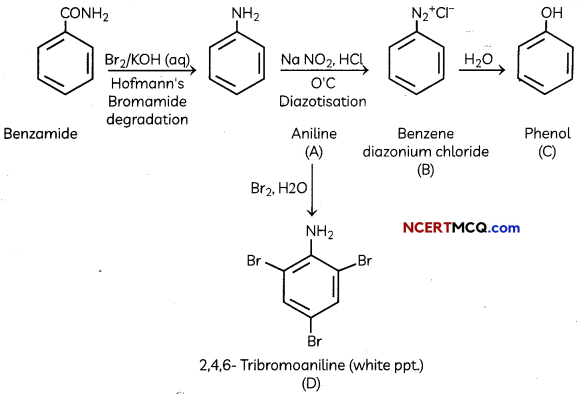SECTION – C
(Section C-Question No 12 is case-based question carrying 5 marks.)

Question 12.
Electrolysis, process by which electric current is passed through a substance to effect a chemical change. The process is carried out in an electrolytic cell, an apparatus consisting of positive and negative electrodes held apart and dipped into a solution containing positively and negatively charged ions. The substance to be transformed may form the electrode, may constitute the solution, or may be dissolved in the solution. Electric current (i.e., electrons) enters through the negatively charged electrode (cathode); components of the solution travel to this electrode, combine with the electrons, and are transformed (reduced). The products can be neutral elements or new molecules. Components of the solution also travel to the other electrode (anode), give up their electrons, and are transformed (oxidized) to neutral elements or new molecules.

Electrolysis is used extensively in metallurgical processes, such as in extraction (electrowinning) or purification (electrorefining) of metals from oresor compounds and in deposition of metals from solution (electroplating). Metallic sodium and chlorine gas are produced by the electrolysis of molten sodium chloride; electrolysis of an aqueous solution of sodium chloride yields sodium hydroxide and chlorine gas. Hydrogen and oxygen are produced by the electrolysis of water.

Michael Faraday discovered in 1833 that there is always a simple relationship between the amount of substance produced or consumed at an electrode during electrolysis and the quantity of electrical charge Q which passes through the cell.
(A) Name two metals which can be purified using electrolytic refining.
(B) What are the electrolysis products of an aqueous solution of sodium chloride?
(C) How much charge in terms of Faraday is required for the reduction of 1 mol of Cu2+ to Cu?
(D) How many moles of mercury will be produced by electrolysing 1.0 M Hg(NO3)2 solution with a current of 2.00 A for 3 hours? [Hg(NO3)2 = 200.6 g mol-1].
OR
A steady current of 2 amperes was passed through two electrolytic cells X and Y connected in series containing electrolytes FeSO4 and ZnSO4 until 2.8 g of Fe deposited at the cathode of cell X. How long did the current flow?
(Molar mass: Fe = 56 g mol-1, Zn = 65.3 g mol-1, 1F = 96500 C mol-1)
(A) Na (Sodium) and K (Potassium)

(B) H2 gas is liberated at cathode. Cl2 gas is liberated at anode. NaOH is formed in the solution.
Explanation:At anode: 2Cl → Cl2 + 2e; At cathode: 2H+ + 2e → H2

(C) Cu2+ + 2e- → Cu
Quantity of charge required for reduction of 1 mol of Cu2+ = 2F(F = Faraday)
Explanation: Faraday’s first law of electrolysis – The amount of any substance deposited or liberated at the electrode is directly proportional to the quantity of electricity passing through the electrolyte.(D) Mass of mercury produced at the cathode,OR
(D) Current i = 2 A, Mass m of Fe = 2.8 g
Molar Mass M of Fe = 56 g mol-1, 1F = 96500 c Fe2+ + 2e” -> Fe (Charge required = 2F)